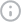0 hidden charges. 0 forex# How to calculate the debt-to-equity ratio?FACT CHECKED
Reviewed by
.
Created on
March 29, 2023## Summary

What’s Inside

The debt-to-equity ratio (DE ratio) is a financial ratio used to evaluate the proportion of a company’s liabilities relative to its shareholders’ equity. If you plan to invest in the stock market over the long term, it’s important to know how to calculate the debt-to-equity ratio from a company's balance sheet.

Investors use the debt-to-equity ratio as a part of evaluating the fundamentals of a company. Calculating is fairly simple because you only need two key metrics — the company’s total liabilities and equity.

## Decoding the Debt-to-Equity Ratio

An important part of learning how to calculate a company's debt-to-equity ratio involves understanding how to decode it. The ratio essentially tells you how much debt a company has relative to its equity. In other words, it shows you how the proportion of debt and equity is used to finance the purchase of a company’s assets.

The lower the debt-to-equity ratio, the better it is. It implies less dependence on debt financing. Experts typically peg the ideal DE ratio at 2:1, although it is advisable to choose stocks of companies with a DE ratio of 1.5 to 2. Anything higher could be a risk because it indicates significantly high debt levels in the company.

## 4 Easy Steps to Calculate the Debt-to-Equity Ratio

If you’re wondering how to calculate a company's debt-to-equity ratio, here are the 4 easy steps you need to follow.

### Identify the Total Shareholders’ Equity

The shareholders’ equity can be found on a company's balance sheet. This includes the share capital and the reserves and surplus. For instance, let’s assume the share capital of a company is ₹50 lakhs, and its reserves and surplus are ₹40 lakhs. So, the total shareholders’ equity will be ₹90 lakhs.

### Identify the Total Debt in the Company’s Books

The total debt includes the long-term and short-term borrowings of a company. For instance, if the long-term borrowings of a company come up to ₹40 lakhs and its short-term borrowings amount to Rs. 60 lakhs, its total debt would be ₹1 crore.

### Substitute the Numbers in the Right Formula

The next important step in calculating the debt-to-equity ratio involves using the right formula. The DE ratio is computed as follows:

DE Ratio = Total Liabilities ÷ Shareholder’s Equity

This formula requires you to substitute the numbers obtained in the previous two steps.

### Calculate the DE Ratio

Using the formula, we get a DE ratio of 1.11 (i.e. Rs. 1 crore ÷ Rs. 90 lakhs). This essentially means the debt of the company is 1.11 times its equity.

## Choose Strong Companies for Your Portfolio

Now that you know how to calculate the debt-to-equity ratio, you can use this financial metric to identify strong companies for your portfolio. This ratio can be crucial for evaluating stocks from domestic and international markets. So, if you want to invest in US stocks, please check the DE ratio before buying.

Fi Money can make it easier for you to purchase and invest in US stocks from India. You can add the stocks of top US companies to your portfolio at industry-best forex rates and zero brokerage fees today.

### What is the debt-equity ratio?

The debt-equity ratio is a financial metric that tells you a company's debt levels as a percentage of its equity. It indicates the proportion of debt and equity used to finance the purchase of the company’s assets.

### What is the formula for debt ratio and an example of how it’s used?

The formula for how calculating the debt-to-equity ratio is as follows. You need to divide the company's total liabilities by its shareholders’ equity. For example, if the total liabilities of a company are Rs. 20 lakhs, and if its shareholders’ equity is Rs. 15 lakhs, the debt-to-equity ratio will be 1.33 (i.e. Rs. 20 lakhs ÷ Rs. 15 lakhs).

### How do you calculate the debt-equity ratio?

To calculate the debt-equity ratio, you must first identify the total debt and the total shareholders’ equity in a company. The next step in how to find the debt-to-equity ratio involves dividing the total liabilities by the total equity. The resulting number is the DE ratio.

# DisclaimerInvestment and securities are subject to market risks. Please read all the related documents carefully before investing. The contents of this article are for informational purposes only, and not to be taken as a recommendation to buy or sell securities, mutual funds, or any other financial products.